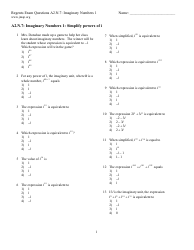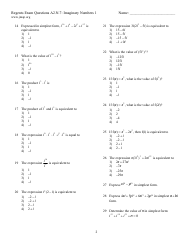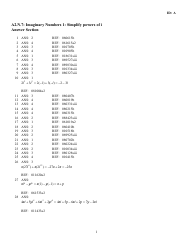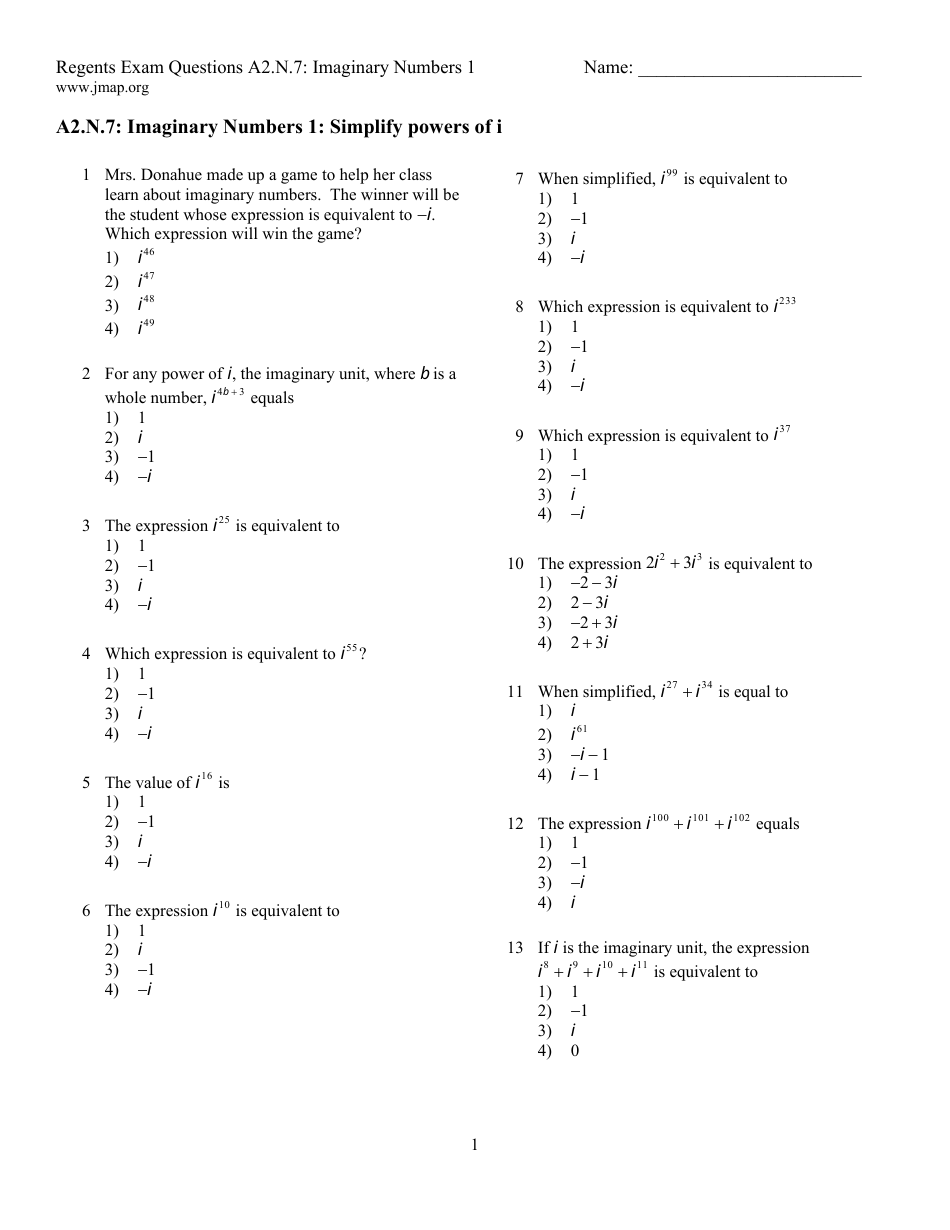# Imaginary Numbers Worksheet With Answer Key

An Imaginary Numbers Worksheet with Answer Key is a learning resource used in math education. It helps students practice and reinforce their understanding of imaginary numbers, which are a mathematical concept involving the square root of negative numbers. The answer key provides solutions to the problems on the worksheet, allowing students to check their work and learn from any mistakes.

## FAQ

Q: What are imaginary numbers?
A: Imaginary numbers are numbers that involve the imaginary unit, denoted by the letter 'i', which is defined as the square root of -1.

Q: What is the square root of -1?
A: The square root of -1 is denoted as 'i', and it is the mathematical representation of an imaginary number.

Q: How are imaginary numbers used in mathematics?
A: Imaginary numbers are used in various branches of mathematics, such as complex numbers, electrical engineering, and physics.

Q: How do you add and subtract imaginary numbers?
A: To add or subtract imaginary numbers, you add or subtract the real parts separately and the imaginary parts separately.

Q: What is the conjugate of an imaginary number?
A: The conjugate of an imaginary number is obtained by changing the sign of its imaginary part. For example, the conjugate of 3i is -3i.

Q: What is the absolute value of an imaginary number?
A: The absolute value (also known as the modulus) of an imaginary number is the distance of the number from the origin on the complex plane. It is always a positive real number.

Q: Can imaginary numbers be squared?
A: Yes, imaginary numbers can be squared. The square of 'i' is -1.

Q: What is the purpose of the imaginary unit in mathematics?
A: The imaginary unit 'i' allows for the representation and manipulation of numbers that do not have real solutions, such as the square root of a negative number.

Q: Are imaginary numbers used in the real world?
A: Yes, imaginary numbers are used in various real-world applications, such as in electrical circuit analysis, signal processing, and quantum mechanics.

Q: Can imaginary numbers be plotted on a number line?
A: No, imaginary numbers cannot be plotted on a number line. They are represented on the complex plane, with the real part on the x-axis and the imaginary part on the y-axis.

•1

•2

•3

•4

•